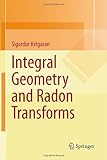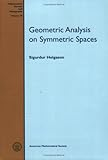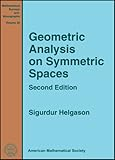search for books and compare prices
Sigurdur Helgason has written 10 work(s)
Search for other authors with the same name
displaying 1 to 10 | at end
show results in order: alphabetically | oldest to newest | newest to oldestProduct Description: In this text, integral geometry deals with Radonâs problem of representing a function on a manifold in terms of its integrals over certain submanifoldsâhence the term the Radon transform. Examples and far-reaching generalizations lead to fundamental problems such as: (i) injectivity, (ii) inversion formulas, (iii) support questions, (iv) applications (e...read more

Hardcover:

9781441960542 | Springer Verlag, November 17, 2010, cover price $79.99 | About this edition: In this text, integral geometry deals with Radonâs problem of representing a function on a manifold in terms of its integrals over certain submanifoldsâhence the term the Radon transform. Paperback: 9781489994202 | Springer Verlag, October 11, 2014, cover price$79.99 | About this edition: In this text, integral geometry deals with Radonâs problem of representing a function on a manifold in terms of its integrals over certain submanifoldsâhence the term the Radon transform.

Product Description: This book gives the first systematic exposition of geometric analysis on Riemannian symmetric spaces and its relationship to the representation theory of Lie groups. The book starts with modern integral geometry for double fibrations and treats several examples in detail...read more

Hardcover:

9780821845301 | Revised edition (Amer Mathematical Society, November 30, 2008), cover price $97.00 | About this edition: This book gives the first systematic exposition of geometric analysis on Riemannian symmetric spaces and its relationship to the representation theory of Lie groups. 9780821815380 | Amer Mathematical Society, July 1, 1994, cover price$54.00 | About this edition: This author gives the first systematic exposition of geometric analysis on Riemannian symmetric spaces and its relationship to the representation theory of semisimple Lie groups.

Product Description: The first edition of this book has been out of print for some time and I have decided to follow the publisher's kind suggestion to prepare a new edition. Many examples with explicit inversion formulas and range theoÂ­ rems have been added, and the group-theoretic viewpoint emphasized...read more

Hardcover:

9780306392054 | Gardners Books, January 1, 2004, cover price $111.15 9780817641092 | 2 sub edition (Birkhauser, August 1, 1999), cover price$89.99 | About this edition: The Radon transform is an important topic in integral geometry which deals with the problem of expressing a function on a manifold in terms of its integrals over certain submanifolds.
9783764330064 | Birkhauser, June 1, 1980, cover price $38.50 | About this edition: The Radon transform is an important topic in integral geometry which deals with the problem of expressing a function on a manifold in terms of its integrals over certain submanifolds. Paperback: 9781475714654 | Springer Verlag, October 9, 2013, cover price$99.00 | About this edition: The first edition of this book has been out of print for some time and I have decided to follow the publisher's kind suggestion to prepare a new edition.

Product Description: The study of homogeneous spaces provides excellent insights into both differential geometry and Lie groups. In geometry, for instance, general theorems and properties will also hold for homogeneous spaces, and will usually be easier to understand and to prove in this setting...read more (view table of contents, read Amazon.com's description)

Hardcover:

9780821828489 | Amer Mathematical Society, August 1, 2001, cover price $84.00 | About this edition: The study of homogeneous spaces provides excellent insights into both differential geometry and Lie groups. 9780123384607 | Academic Pr, December 1, 1978, cover price$69.95 | also contains Environment of Care Sample Annual Evaluation And Report to Leadership | About this edition: The present book is intended as a textbook and reference work on three topics in the title.

Product Description: This volume, the second of Helgason's impressive three books on Lie groups and the geometry and analysis of symmetric spaces, is an introduction to group-theoretic methods in analysis on spaces with a group action. The first chapter deals with the three two-dimensional spaces of constant curvature, requiring only elementary methods and no Lie theory...read more (view table of contents, read Amazon.com's description)

Hardcover:

9780821826737 | Amer Mathematical Society, November 1, 2000, cover price $69.00 | About this edition: This volume, the second of Helgason's impressive three books on Lie groups and the geometry and analysis of symmetric spaces, is an introduction to group-theoretic methods in analysis on spaces with a group action. 9780123383013 | Academic Pr, June 1, 1984, cover price$67.00 | also contains Tiger Woods Pga Tour '07: Prima Official Strategy Guide | About this edition: The present book is intended as a textbook and reference work on three topics in the title.

Product Description: Sigurdur Helgason's Differential Geometry and Symmetric Spaces was quickly recognized as a remarkable and important book. For many years, it was the standard text both for Riemannian geometry and for the analysis and geometry of symmetric spaces...read more (view table of contents, read Amazon.com's description)

Hardcover:

9780821827352 | Amer Mathematical Society, November 1, 2000, cover price $57.00 | About this edition: Sigurdur Helgason's Differential Geometry and Symmetric Spaces was quickly recognized as a remarkable and important book. Product Description: The theme of this volume is a treatment of differential equations on a$C^\infty$manifold$V$by separation of variables techniques. More specifically, given a Lie transformation group$L$of$V$and a Lie subgroup$H\subset L$, if$D(V)$is the set of differential operators on$V$invariant under$L$, then the principal object of study is the set of distributions$T$on$V$satisfying the following two conditions:(i)$T$is an eigendistribution of each$D\in D(V)$; (ii)$T$is invariant under$H$...read more Paperback: 9780821816646 | Amer Mathematical Society, June 1, 1982, cover price$28.00 | About this edition: The theme of this volume is a treatment of differential equations on a $C^\infty$ manifold $V$ by separation of variables techniques.

Product Description: This book deals with harmonic analysis on the complete simply connected two-dimensional manifolds of constant curvature (the Euclidean plane, the two-sphere and the non-Euclidean plane). The treatment is elementary and Lie group theory is not required...read more

Hardcover:

9783764330514 | Birkhauser, June 1, 1981, cover price $59.95 | About this edition: This book deals with harmonic analysis on the complete simply connected two-dimensional manifolds of constant curvature (the Euclidean plane, the two-sphere and the non-Euclidean plane). Product Description: Concentrating on complete simply connected two-dimensional manifolds with constant curvature, Topics in Harmonic Analysis on Homogeneous Spaces is a brief and elementary presentation of the subject. Treating the results of recent work in this highly active field, the author offers numerous proofs illustrating harmonic analysis on symmetric spaces...read more Hardcover: 9780817630515 | Gardners Books, January 1, 1981, cover price$30.10 | About this edition: Concentrating on complete simply connected two-dimensional manifolds with constant curvature, Topics in Harmonic Analysis on Homogeneous Spaces is a brief and elementary presentation of the subject.

displaying 1 to 10 | at end